Students can Download Tamil Nadu 12th Physics Model Question Paper 5 English Medium Pdf, Tamil Nadu 12th Physics Model Question Papers helps you to revise the complete Tamilnadu State Board New Syllabus, helps students complete homework assignments and to score high marks in board exams.

## TN State Board 12th Physics Model Question Paper 5 English Medium

General Instructions:

• The question paper comprises of four parts.
• You are to attempt all the parts. An internal choice of questions is provided wherever applicable.
• All questions of Part I, II, III, and IV are to be attempted separately.
• Question numbers 1 to 15 in Part I are Multiple Choice Questions of one mark each.
These are to be answered by choosing the most suitable answer from the given four
alternatives and writing the option code and the corresponding answer
• Question numbers 16 to 24 in Part II are two-mark questions. These are to be answered
in about one or two sentences.
• Question numbers 25 to 33 in Part III are three-mark questions. These are to be answered
in about three to five short sentences.
• Question numbers 34 to 38 in Part IV are five-mark questions. These are to be answered
in detaiL Draw diagrams wherever necessary.

Time: 3 Hours
Max Marks: 70

Part – I

Answer all the questions. Choose the correct answer. [15 x 1 = 15]

Question 1.
If voltage applied on a capacitor is increased from V to 2V, Choose the correct conclusion,
(a) Q remains the same, C is doubled
(b) Q is doubled, C doubled
(c) C remains same, Q doubled
(d) Both Q and C remain same
(c) C remains same, Q doubled

Question 2.
The electric field in the region between two concentric charged spherical shells
(a) is zero
(b) increases with distance from centre
(c) is constant
(d) decreases with distance from centre
(d) decreases with distance from centreQuestion 3.
In India electricity is supplied for domestic use at 220 V. It is supplied at 110 V in USA. If the resistance of a 60W bulb for use in India is R, the resistance of a 60W bulb for use in USA will be …………
(a) R
(b) 2R
(c) $$\frac{\mathbf{R}}{4}$$
(d) $$\frac{\mathbf{R}}{2}$$
(c) $$\frac{\mathbf{R}}{4}$$

Question 4.
The vertical component of Earth’s magnetic field at a place is equal to the horizontal component. What is the value of angle of dip at this place?
(a) 30°
(b) 45°
(c) 60°
(d) 90°
(b) 45°

Question 5.
A step-down transformer reduces the supply voltage from 220 V to 11 V and increase the current from 6 A to 100 A. Then its efficiency is
(a) 1.2
(b) 0.83
(c) 0.12
(d) 0.9
(b) 0.83

Question 6.
Faraday’s law of electromagnetic induction is related to the …………….
(a) Law of conservation of charge
(b) Law of conservation of energy
(c) Third law of motion
(d) Law of conservation of angular momentum
(b) Law of conservation of energyQuestion 7.
The dimension of $$\frac{1}{\mu_{0} \varepsilon_{0}}$$ is ……….
(a) [L T-1]
(b) [L2 T-2]
(c) [L-1 T]
(d) [L-2 T2]
(b) [L2 T-2]

Question 8.
In a Young’s double-slit experiment, the slit separation is doubled. To maintain the same fringe spacing on the screen, the screen-to-slit distance D must be changed to,…………..
(a) 2D
(b) $$\frac{\mathrm{D}}{2}$$
(c) $$\sqrt{2} \mathrm{D}$$
(d) $$\frac{\mathrm{D}}{\sqrt{2}}$$
(a) 2D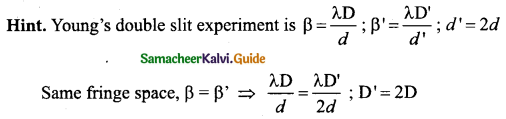Question 9.
The speed of light in an isotropic medium depends on,
(a) its intensity
(b) its wavelength
(c) the nature of propagation
(d) the motion of the source w.r.to medium
(b) its wavelength

Question 10.
The mean wavelength of light from sun is taken to be 550 nm and its mean power is 3.8 x 1026 W. The number of photons received by the human eye per second on the average from sunlight is of the order of ……………..
(a) 1045
(b) 1042
(c) 1054
(d) 1051
(a) 1045
Hint: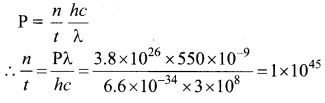Question 11.
As the intensity of incident light increases
(a) kinetic energy of emitted photoelectrons increases
(b) photoelectric current decreases
(c) photoelectric current increases
(d) kinetic energy of emitted photoelectrons decreases
(c) photoelectric current increases
Hint: As the intensity of incident light increases, photoelectric current increases.

Question 12.
In J.J. Thomson e/m experiment, a beam of electron is replaced by that of muons (particle with same charge as that of electrons but mass 208 times that of electrons). No deflection condition is achieved only if
(a) B is increased by 208 times
(b) B is decreased by 208 times
(c) B is increased by 14.4 times
(d) B is decreased by 14.4 times
(c) B is increased by 14.4 times
Hint: In the condition of no deflection $$\frac{e}{m}=\frac{\mathrm{E}^{2}}{2 v \mathrm{B}^{2}}$$
If m is increased by 208 times then B should be increased $$\sqrt{208}$$ = 14.4 times

Question 13.
A forward biased diode is treated as
(a) An open switch with infinite resistance
(b) A closed switch with a voltage drop of 0V
(c) A closed switch in series with a battery voltage of 0.7V
(d) A closed switch in series with a small resistance and a battery.
(d) A closed switch in series with a small resistance and a battery.

Question 14.
The variation of frequency of carrier wave with respect to the amplitude of the modulating
signal is called …………
(a) Amplitude modulation
(b) Frequency modulation
(c) Phase modulation
(d) Pulse width modulation
(b) Frequency modulation

Question 15.
Who is the father of the modem robotics industry formed the world’s first robotic company in 1956?
(a) Joliot
(b) Cormark
(c) Engelberger
(d) Edward purcell
(c) Engelberger

Part – II

Answer any six questions in which Q. No 17 is compulsory.

Question 16.
What is the general definition of electric dipole moment?
The electric dipole moment vector lies along the line joining two charges and is directed from  – q to +q. The SI unit of dipole moment is coulomb meter (cm).
$$\vec{p}=q a \hat{i}-q a(-\hat{i})=2 q a \hat{i}$$Question 17.
Calculate the electric flux through the rectangle of sides 5 cm and 10 cm kept in the region of a uniform electric field 100 NC-1. The angle 0 is 60°. Suppose 0 becomes zero, what is the electric flux?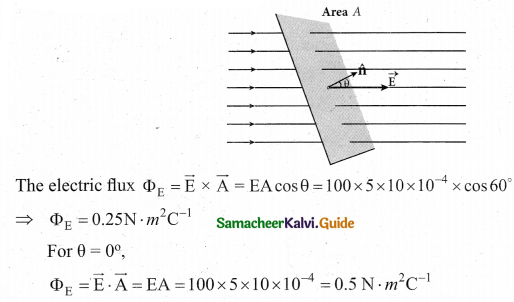Question 18.
What is meant by hysteresis?
The phenomenon of lagging of magnetic induction behind the magnetising field is called hysteresis. Hysteresis means Tagging behind’.

Question 19.
What is meant by wattles current?
The component of current (IpMS sin φ), which has a phase angle of $$\frac{\pi}{2}$$ with the voltage is called reactive component. The power consumed is zero. So that it is also known as ‘Wattless’ current.

Question 20.
What is Snell’s window?
When light entering the water from outside is seen from inside the water, the view is restricted to a particular angle equal to the critical angle ic. The restricted illuminated circular area is called Snell’s window.Question 21.
Define work function of a metal. Give its unit.
The minimum energy needed for an electron to escape from the metal surface is called work function of that metal. It’s unit is electron volt (eV).

Question 22.
The phenomenon of spontaneous emission of highly penetrating radiations such as α, β and γ rays by an element is called radioactivity.

Question 23.
What is the angular momentum of an electron in the third orbit of an atom?
Here n = 3; h = 6.6 x 10-34 Js
Angular momentum.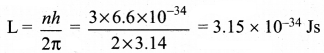Question 24.
Explain the current flow in a NPN transistor

• The conventional flow of current is based on the direction of the motion of holes
• In NPN transistor, current enters from the base into the emitter.

Part – III

Answer any six questions in which Q.No. 27 is compulsory. [6×3 = 18]

Question 25.
Define ‘capacitance’. Give its unit.
The capacitance C of a capacitor is defined as the ratio of the magnitude of charge on either of the conductor plates to the potential difference existing between the conductors.
$$C=\frac{Q}{V} \text { or } Q \propto V$$
The SI unit of capacitance is coulomb per volt or farad (F).Question 26.
Distinguish between drift velocity and mobility

 SNo. Drift Velocity Mobility 1. The drift velocity is the average velocity acquired by the electrons inside the conductor when it is subjected to an electric field. Mobility of an electron is defined as the magnitude of the drift velocity per unit electric field. 2. $$\overrightarrow{\mathrm{V}}_{d}=\vec{a} \tau$$ $$\mu=\frac{e \tau}{m} \text { or } \mu=\frac{\left|\overrightarrow{\mathrm{V}}_ {d}\right|}{\overrightarrow{\mathrm{E}}}$$ 3. It’s unit is ms-1. It’s unit is m2 v_1 s_1

Question 27.
A coil of a tangent galvanometer of diametre 0.24 m has 100 turns. If the horizontal component of Earth’s magnetic field is 25 x 10-6 T then, calculate the current which gives a deflection of 60°.
The diameter of the coil is 0.24 m. Therefore, radius of the coil is 0.12 m.
Number of turns is 100 turns. Earth’s magnetic field is 25 x 10-6 T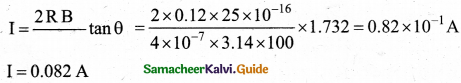Question 28.
Mention the ways of producing induced emf.
Induced emf can be produced by changing magnetic flux in any of the following ways:

• By changing the magnetic field B
• By changing the area A of the coil and
• By changing the relative orientation 0 of the coil with magnetic field

Question 29.
A 400 mH coil of negligible resistance is connected to an AC circuit in which an effective current of 6 mA is flowing. Find out the voltage across the coil if the frequency is 1000 Hz.
L = 400 x 10-3 H; Ieff = 6 x 10-3A; f= 1000 Hz
Inductive reactance, XL = Lω = L x 2πf = 2 x 3.14 x 1000 x 0.4 = 2512Ω
Voltage across L, V – IXL = 6 x 10-3 x 2512 =15.072 V (RMS)Question 30.
What are the sign conventions followed for lenses?
The sign conventions for thin lenses differ only in the signs followed for focal lengths.
(a) The sign of focal length is not decided on the direction of measurement of the focal length from the pole of the lens as they have two focal lengths, one to the left and another to the right (primary and secondary focal lengths on either side of the lens).

(b) The focal length of the thin lens is taken as positive for a converging lens and negative for a diverging lens.

Question 31.
Write down the draw backs of Bohr atom’model.
Limitations of Bohr atom model
The following are the drawbacks of Bohr atom model

• Bohr atom model is valid only for hydrogen atom or hydrogen like-atoms but not for
complex atoms.
• When the spectral lines are closely examined, individual lines of hydrogen spectrum is
accompanied by a number of faint lines. These are often called fine structure. This is not explained by Bohr atom model.
• Bohr atom model fails to explain the intensity variations in the spectral lines.
• The distribution of electrons in atoms is not completely explained by Bohr atom model.

Question 32.
What do you mean by leakage current in a diode?
The leakage current in a diode is the current that the diode will leak when a reverse voltage is applied to it. Under the reverse bias, a very small current in μA, flows across the junction. This is due to the flow of the minority charge carriers called the leakage current or reverse saturation current.

Question 33.
Distinguish between wireline and wireless communication.

 Wireline communication Wireless communication It is a point-to-point communication. It is a broadcast mode communication. It uses mediums like wires, cable and optical fibres. It uses free space as a communication medium. These systems cannot be used for long distance transmission as they are connected. These systems can be used for long distance transmission. Ex. telephone, intercom and cable TV. Ex. mobile, radio or TV broadcasting and satellite communication.

Part – IV

Answer all the questions. [5 x 5 = 25]

Question 34.
(a) Derive an expression for the torque experienced by a dipole due to a uniform electric field.
Torque experienced by an electric dipole in the uniform electric field: Consider an electric dipole of dipole moment $$\vec{p}$$ placed in a uniform electric field $$\vec{E}$$ whose field lines are equally spaced and point in the same direction. The charge +q will experience a force q$$\vec{E}$$  in the direction of the field and charge -q will experience a force -q $$\vec{E}$$ in a direction opposite to the field. Since the external field $$\vec{E}$$ is uniform, the total force acting on the dipole is zero. These two forces acting at different points will constitute a couple and the dipole experience a torque. This torque tends to rotate the dipole. (Note that electric field lines of a uniform field are equally spaced and point in the same direction). The total torque on the dipole about the point O.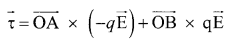Using right-hand corkscrew rule, it is found that total torque is perpendicular to the plane of the paper and is directed into it.
The magnitude of the total torque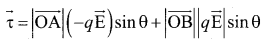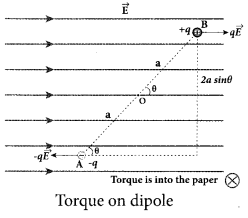where θ is the angle made by $$\vec{p} \text { with } \overrightarrow{\mathrm{E}}$$ .
Since p = 2aq, the torque is written in terms of the vector product as $$\vec{\tau}=\vec{p} \times \vec{E}$$
The magnitude of this torque is τ = pE sin θ and is maximum
when θ =90°.
This torque tends to rotate the dipole and align it with the electric field [/latex]\overrightarrow{\mathrm{E}}[/latex] . Once $$\overrightarrow{\mathrm{p}}$$ is aligned with $$\overrightarrow{\mathrm{E}}$$, the total torque on the dipole becomes zero.[OR]

(b) Explain the equivalent resistance of a parallel resistor network.
Resistors in parallel: Resistors are in parallel when they are connected across the same potential difference as shown in fig. (a).
In this case, the total current I that leaves the battery in split into three separate paths. Let I1, I2 and I3 be the current through the resistors R1 ,R2 and R3 respectively. Due to the conservation of charge, total current in the circuit I is equal to sum of the currents through each of the three resistors.
I = I1 + I2 + I3 ………………… (1)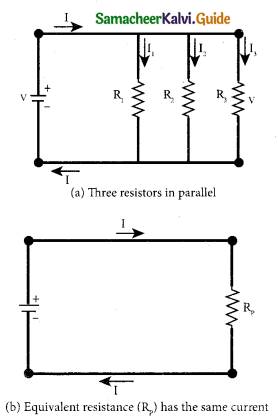Since the voltage across each resistor is the same, applying Ohm’s law to each resistor, we have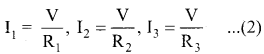Substituting these values in equation (1) we get.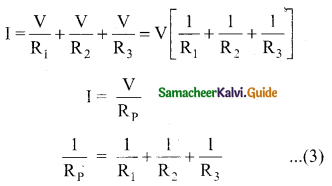Here Rp is the equivalent resistance of the parallel combination of the resistors. Thus, when a number of resistors are connected in parallel, the sum of the reciprocal of the values of resistance of the individual resistor is equal to the reciprocal of the effective resistance of the combination as shown in the fig. (b).
Note: The value of equivalent resistance in parallel connection will be lesser than each individual resistance.

Question 35.
(a) Obtain a relation for the magnetic induction at a point along the axis of a circular coil ‘ carrying current.
Magnetic field produced along the axis of the current carrying circular coil: Consider a current carrying circular loop of radius R and let I be the current flowing through the wire in the direction. The magnetic field at a point P on the axis of the circular coil at a distance z from its center of the coil O. It is computed by taking two diametrically opposite line elements of the coil each of length $$d \bar{l}$$ at C and D Let r be the vector joining the current element (I $$d \bar{l}$$) at C to the point P.
PC = PD = r = $$r=\sqrt{\mathrm{R}^{2}+\mathrm{Z}^{2}}$$
PC = PD = r = $$r=\sqrt{\mathrm{R}^{2}+\mathrm{Z}^{2}}$$
angle ∠CPO = ∠DPO = θ
According to Biot-Savart’s law, the magnetic field at P due to the current element $$d \bar{l}$$ is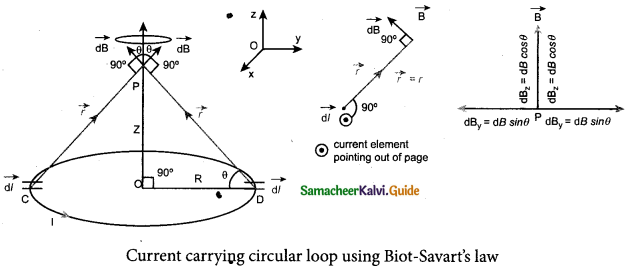The magnitude of magnetic field due to current element I $$d \bar{l}$$ at C and D are equal because of equal distance from the coil. The magnetic field dB due to each current element I dl is resolved into two components; dB sin θ along y-direction and dB cos θ along the z-direction. Horizontal components of each current element cancels out while the vertical components (dB cos θ $$\hat{k}$$ ) alone contribute to total magnetic field at the point P.
If we integrate $$d \bar{l}$$ around the loop, $$d \bar{B}$$ sweeps out a cone, then the net magnetic field $$\overrightarrow{\mathrm{B}}$$ at point P is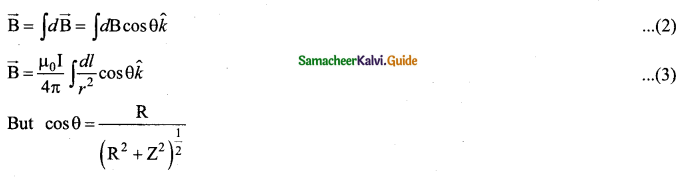Using Pythagorous theorem r2 = R2 + Z2 and integrating line element from 0 to 2πR, we get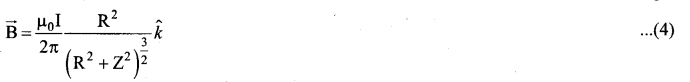Note that the magnetic field $$\bar{B}$$ points along the direction from the point O to P. Suppose if the current flows in clockwise direction, then magnetic field points in the direction from the point P to O.[OR]

(b) Explain the working of a single-phase AC generator with necessary diagram.
Single phase AC generator: In a single phase AC generator, the armature conductors are connected in series so as to form a single circuit which generates a single-phase alternating emf and hence it is called single-phase alternator.

The simplified version of a AC generator is discussed hire. Consider a stator core consisting of 2 slots in which 2 armature conductors PQ and RS are mounted to form single-turn rectangular loop PQRS. Rotor has 2 salient poles with field windings which can be magnetized by means of DC source.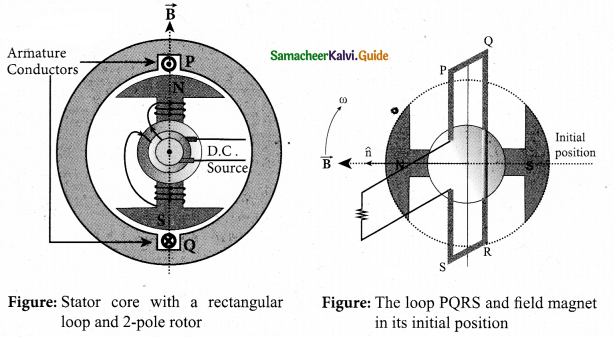Working: The loop PQRS is stationary and is perpendicular to the plane of the paper. When field windings are excited, magnetic field is produced around it. The direction of magnetic field passing through the armature core. Let the field magnet be rotated in clockwise direction by the prime mover. The axis of rotation is perpendicular to the plane of the paper.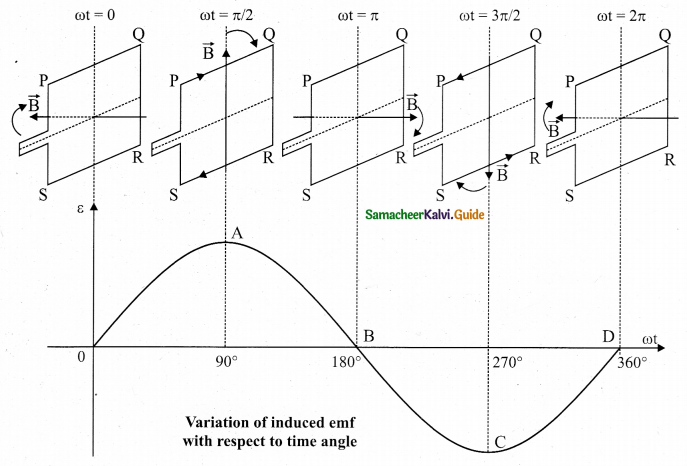Assume that initial position of the field magnet is horizontal. At that instant, the direction of magnetic field is perpendicular to the plane of the loop PQRS. The induced emf is zero. This is represented by origin O in the graph between induced emf and time angle.

When field magnet rotates through 90°, magnetic field becomes parallel to PQRS. The induced emfs across PQ and RS would become maximum. Since they are connected in series, emfs are added up and the direction of total induced emf is given by Fleming’s right hand rule.

Care has to be taken while applying this rule; the thumb indicates the direction of the motion of the conductor with respect to field. For clockwise rotating poles, the conductor appears to be rotating anti-clockwise. Flence, thumb should point to the left. The direction of the induced emf is at right angles to the plane of the paper. For PQ, it is downwards and for RS upwards. Therefore, the current flows along PQRS. The point A in the graph represents this maximum emf.

For the rotation of 180° from the initial position, the field is again perpendicular to PQRS and the induced emf becomes zero. This is represented by point B. The field magnet becomes again parallel to PQRS for 270° rotation of field magnet. The induced emf is maximum but the direction is reversed. Thus the current flows along SRQP. This is represented by point C.

On completion of 360°, the induced emf becomes zero and is represented by the point D. From the graph, it is clear that emf induced in PQRS is alternating in nature.

Therefore, when field magnet completes one rotation, induced emf in PQRS finishes one cycle. For this construction, the frequency of the induced emf depends on the speed at which the field magnet rotates.

Question 36.
(a) What is emission spectra? Give their types.
Emission spectra: When the spectrum of self luminous source is taken, we get emission spectrum. Each source has its own characteristic emission spectrum. The emission spectrum _ can be divided into three types:

(i) Continuous emission spectra (or continuous spectra): If the light from incandescent lamp filament bulb) is allowed to pass through prism (simplest spectroscope), it splits into seven colours. Thus, it consists of wavelengths containing all the visible colours ranging from violet to red. Examples: spectrum obtained from carbon arc, incandescent solids, liquids gives continuous spectra.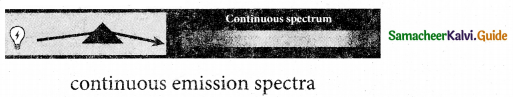(ii) Line emission spectrum (or line spectrum): Suppose light from hot gas is allowed to pass through prism, line spectrum is observed. Line spectra are also known as discontinuous spectra. The line spectra are sharp lines of definite wavelengths or frequencies. Such spectra arise due to excited atoms of elements. These lines are the characteristics of the element which means it is different for different elements. Examples: spectra of atomic hydrogen, helium, etc.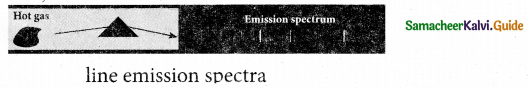(iii) Band emission spectrum (or band spectrum): Band spectrum consists of several number of very closely spaced spectral lines which overlapped together forming specific bands which are separated by dark spaces, known as band spectra. This spectrum has a sharp edge at one end and fades out at the other end. Such spectra arise when the molecules are excited. Band spectrum is the characteristic of the molecule hence, the structure of the molecules can be studied using their band spectra. Examples, spectra of hydrogen gas, ammonia gas in the discharge tube etc.[Or]

(b) Describe the Fizeau’s method to determine speed of light.
Fizeau’s method to determine speed of light:
Apparatus: The light from the source S was first allowed to fall on a partially silvered glass plate G kept at an angle of 45° to the incident light from the source. The light then was allowed to pass through a rotating toothed-wheel with N teeth and N cuts of equal widths whose speed of rotation could be varied through an external mechanism.

The light passing through one cut in the wheel will, get reflected by a mirror M kept at a long distance d, about 8 km from the toothed wheel. If the toothed wheel was not rotating, the reflected light from the mirror would again pass through the same cut and reach the eyes of the observer through the partially silvered glass plate.

Working: The angular speed c rotation of the toothed wheel was increased from zero to a value co until light passing through one cut would completely be blocked by the adjacent tooth. This is ensured by the disappearance of light while looking through the partially silvered glass plate.

Expression for speed of light: The speed of light in air v is equal to the ratio of the distance the light travelled from the toothed wheel to the mirror and back 2d to the time taken t.
$$v=\frac{2 d}{t}$$ ………………… (1)

The distance d is a known value from the arrangement. The time taken t for the light to travel the distance to and fro is calculated from the angular speed ω of the toothed wheel. The angular speed ω of the toothed wheel when the light disappeared for the first time is,
$$\omega=\frac{\theta}{t}$$ ………………….. (2)

Here, θ is the angle between the tooth and the slot which is rotated by the toothed wheel within that time t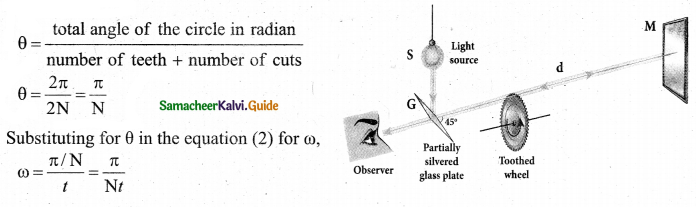Rewriting the above equation for t
$$t=\frac{\pi}{\mathrm{N} \omega}$$ ……………….. (3)
Substituting t from equation (3) in equation (1)
$$v=\frac{2 d}{\pi / \mathrm{N} \omega}$$
$$v=\frac{2 d \mathrm{N} \omega}{\pi}$$ …………….(4)
Fizeau had some difficulty to visually estimate the minimum intensity blocked by the adjacent tooth, and his value for speed of light was very value.

Question 37.
(a) List out the laws of photoelectric effect.
Laws of photoelectric effect:

• For a given frequency of incident light, the number of photoelectrons emitted is directly proportional to the intensity of the incident light. The saturation current is also directly proportional to the intensity of incident light.
• Maximum kinetic energy of the photo electrons is independent of intensity of the incident light.
• Maximum kinetic energy of the photo electrons from a given metal is directly proportional to the frequency of incident light.
• For a given surface, the emission of photoelectrons takes place only if the frequency of incident light is greater than a certain minimum frequency called the threshold frequency.
• There is no time lag between incidence of light and ejection of photoelectrons.[OR]

(b) Describe the working of nuclear reactor with a block diagram.
Nuclear reactor:

• Nuclear reactor is a system in which the nuclear fission takes place in a self-sustained controlled manner and the energy produced is used either for research purpose or for power generation.
• The main parts of a nuclear reactor are fuel, moderator and control rods. In addition to this, there is a cooling system which is connected with power generation set up.

Fuel:

• The fuel is fissionable material, usually uranium or plutonium. Naturally occurring uranium contains only $$0.7 \% \text { of }_{92}^{235} \mathrm{U} \text { and } 99.3 \% \text { are only }^{238} \mathrm{g}_{2} \mathrm{U}$$ . So the $$_{ 92 }^{ 235 }{ U }$$ must be enriched such that it contains at least 2 to 4% of $$_{ 92 }^{ 235 }{ U }$$ .
• In addition to this, a neutron source is required to initiate the chain reaction for the first time. A mixture of beryllium with plutonium or polonium is used as the neutron source. During fission of $$_{ 92 }^{ 235 }{ U }$$, only fast neutrons are emitted but the probability of initiating fission by it in another nucleus is very low. Therefore, slow neutrons are preferred for sustained nuclear reactions.

Moderators:

• The moderator is a material used to convert fast neutrons into slow neutrons. Usually the moderators are chosen in such a way that it must be very light nucleus having mass comparable to that of neutrons. Hence, these light nuclei undergo collision with fast neutrons and the speed of the neutron is reduced
• Most of the reactors use water, heavy water (D20) and graphite as moderators. The blocks of uranium stacked together with blocks of graphite (the moderator) to form a large pile.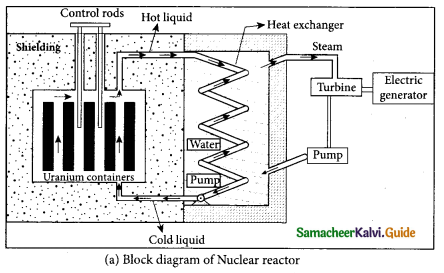Control rods:

• The control rods are used to adjust the reaction rate. During each fission, on an average 2.5 neutrons are emitted and in order to have the controlled chain reactions, only one neutron is allowed to cause another fission and the remaining neutrons are absorbed by the control rods.
• Usually cadmium or boron acts as control rod material and these rods are inserted into the uranium blocks. Depending on the insertion depth of control rod into the uranium, the average number of neutrons produced per fission is set to be equal to one or greater than one.
• If the average number of neutrons produced per fission is equal to one, then reactor is said to be in critical state. In fact, all the nuclear reactors are maintained in critical state by suitable adjustment of control rods. If it is greater than one, then reactor is said to be in super-critical and it may explode sooner or may cause massive destruction.

Shielding:

• For a protection against harmful radiations, the nuclear reactor is surrounded by a concrete wall of thickness of about 2 to 2.5 m.Cooling system:

• The cooling system removes the heat generated in the reactor core. Ordinary water, heavy water and liquid sodium are used as coolant since they have very high specific heat capacity and have large boiling point under high pressure.
• This coolant passes through the fuel block and carries away the heat to the steam generator through heat exchanger. The steam runs the turbines which produces electricity in power reactors.

Question 38.
(a) Explain the working principle of a solar cell. Mention its applications.
Solar cell:
A solar cell, also known as photovoltaic cell, converts light energy directly into electricity or electric potential difference by photovoltaic effect. It is basically a p-n junction which generates emf when solar radiation falls on the p-n junction. A solar cell is of two types: p-type and n-type.

Both types use a combination of p-type and n-type Silicon which together forms the p-n junction of the solar cell. The difference is that p-type solar cells use p-type Silicon as the base with an ultra-thin layer of n-type Silicon as shown in Figure, while n-type solar cell uses the opposite combination. The other side of the p-Silicon is coated with metal which forms the back electrical contact. On top of the n-type Silicon, metal grid is deposited which acts as the front electrical contact. The top of the solar cell is coated with anti-reflection coating and toughened glass.

In a solar cell, electron-hole pairs are generated due to the absorption of light near the junction. Then the charge carriers are separated due to the electric field of the depletion region. Electrons move towards n-type Silicon and holes move towards p-type Silicon layer.

The electrons reaching the n-side are collected by the front contact and holes reaching p-side are collected by the back electrical contact. Thus a potential difference is developed across solar cell. When an external load is connected to the solar cell, photocurrent flows through the load.

Many solar cells are connected together either in series or in parallel combination to form solar panel or module. Many solar panels are connected with each other to form solar arrays. For  high power applications, solar panels and solar arrays are used.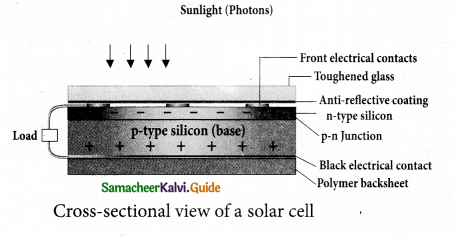Applications:

• Solar cells are widely used in calculators, watches, toys, portable power supplies, etc.
• Solar cells are used in satellites and space applications
• Solar panels are used to generate electricity.

(b) Elaborate on the basic elements of communication system with the necessary block diagram.
Elements of an electronic communication system
Information (Baseband or input signal): Information can be in the form of a sound signal like speech, music, pictures, or computer data which is given as input to the input transducer.

Input transducer
A transducer is a device that converts variations in a physical quantity (pressure, temperature, sound) into an equivalent electrical signal or vice versa. In communication system, the transducer converts the information which is in the form of sound, music, pictures or computer data into corresponding electrical signals. The electrical equivalent of the original information is called the baseband signal. The best example for the transducer is the microphone that converts sound energy into electrical energy.Transmitter:
It feeds the electrical signal from the transducer to the communication channel. It consists of circuits such as amplifier, oscillator, modulator, and power amplifier. The transmitter is located at the broadcasting station.

Amplifier:
The transducer output is very weak and is amplified by the amplifier. Oscillator: It generates high-frequency carrier wave (a sinusoidal wave) for long distance transmission into space. As the energy of a wave is proportional to its frequency, the carrier wave has very high energy.

Modulator:
It superimposes the baseband signal onto the carrier signal and generates the modulated signal.

Power amplifier:
It increases the power level of the electrical signal in order to cover a large distance.

Transmitting antenna:
It radiates the radio signal into space in all directions. It travels in the form of electromagnetic waves with the velocity of light (3 x 108 ms-1).

Communication channel:
Communication channel is used to carry the electrical signal from transmitter to receiver with less noise or distortion. The communication medium is basically of two types: wireline communication and wireless communication.

Noise:
It is the undesirable electrical signal that interfaces with the transmitted signal. Noise attenuates or reduces the quality of the transmitted signal. It may be man-made (automobiles, welding machines, electric motors etc .) or natural (lightening, radiation from sun and stars and environmental effects). Noise cannot be completely eliminated. However, it can be reduced using various techniques.